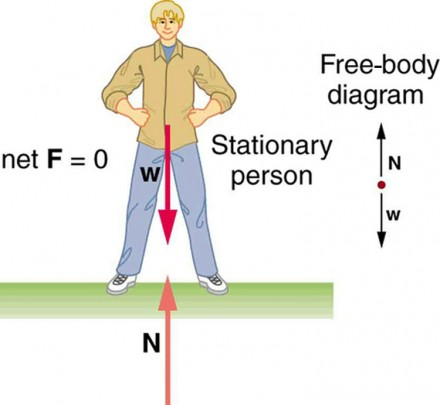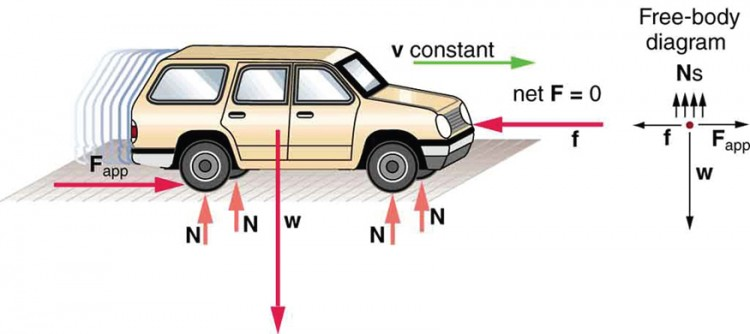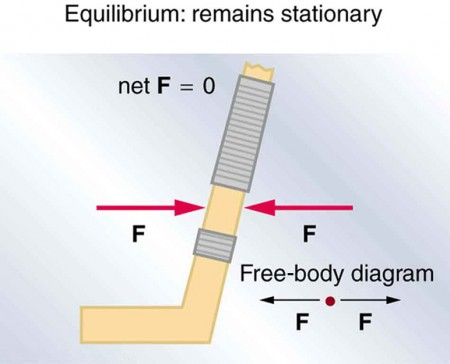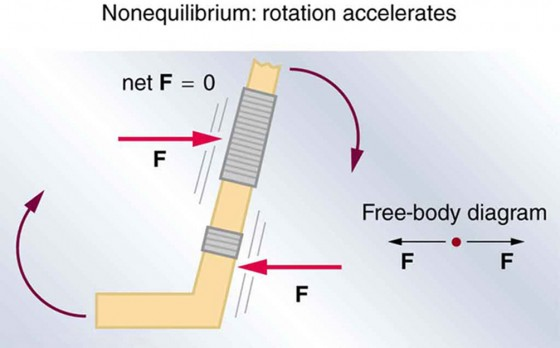## The First Condition for Equilibrium

### Learning Objectives

By the end of this section, you will be able to:

• State the first condition of equilibrium.
• Explain static equilibrium.
• Explain dynamic equilibrium.

The first condition necessary to achieve equilibrium is the one already mentioned: the net external force on the system must be zero. Expressed as an equation, this is simply

net F = 0

Note that if net F is zero, then the net external force in any direction is zero. For example, the net external forces along the typical x– and y-axes are zero. This is written as

net Fx = 0 and Fy = 0

Figure 2 and Figure 3 illustrate situations where net F = 0. for both static equilibrium (motionless), and dynamic equilibrium (constant velocity).Figure 2. This motionless person is in static equilibrium. The forces acting on him add up to zero. Both forces are vertical in this case.Figure 3. This car is in dynamic equilibrium because it is moving at constant velocity. There are horizontal and vertical forces, but the net external force in any direction is zero. The applied force Fapp between the tires and the road is balanced by air friction, and the weight of the car is supported by the normal forces, here shown to be equal for all four tires.

However, it is not sufficient for the net external force of a system to be zero for a system to be in equilibrium. Consider the two situations illustrated in Figure 4 and Figure 5 where forces are applied to an ice hockey stick lying flat on ice. The net external force is zero in both situations shown in the figure; but in one case, equilibrium is achieved, whereas in the other, it is not. In Figure 4, the ice hockey stick remains motionless. But in Figure 5, with the same forces applied in different places, the stick experiences accelerated rotation. Therefore, we know that the point at which a force is applied is another factor in determining whether or not equilibrium is achieved. This will be explored further in the next section.Figure 4. An ice hockey stick lying flat on ice with two equal and opposite horizontal forces applied to it. Friction is negligible, and the gravitational force is balanced by the support of the ice (a normal force). Thus net F = 0. Equilibrium is achieved, which is static equilibrium in this case.Figure 5. The same forces are applied at other points and the stick rotates—in fact, it experiences an accelerated rotation. Here net F = 0 but the system is not at equilibrium. Hence, the net F = 0 is a necessary—but not sufficient—condition for achieving equilibrium.

## PhET Explorations: Torque

Investigate how torque causes an object to rotate. Discover the relationships between angular acceleration, moment of inertia, angular momentum and torque.

## Section Summary

• Statics is the study of forces in equilibrium.
• Two conditions must be met to achieve equilibrium, which is defined to be motion without linear or rotational acceleration.
• The first condition necessary to achieve equilibrium is that the net external force on the system must be zero, so that F = 0.

### Conceptual Questions

1. What can you say about the velocity of a moving body that is in dynamic equilibrium? Draw a sketch of such a body using clearly labeled arrows to represent all external forces on the body.

2. Under what conditions can a rotating body be in equilibrium? Give an example.

## Glossary

static equilibrium:
a state of equilibrium in which the net external force and torque acting on a system is zero
dynamic equilibrium:
a state of equilibrium in which the net external force and torque on a system moving with constant velocity are zero